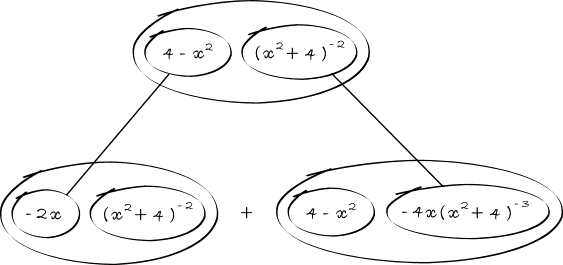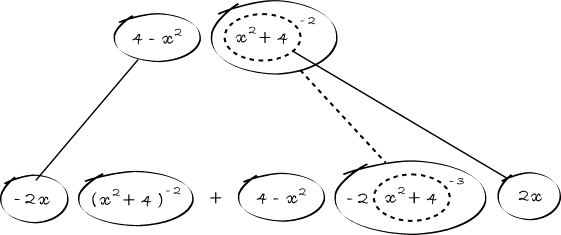# Thread: Locate Inflection Points

1. ## Locate Inflection Points

f(x) = (x)/[(x^2 + 4)]
Locate all inflection points

I got f'(x) = (-x^2 + 4)/[(x^2 +4)]^2
and found f''(x) = [2x(x^4 - 8x^2 - 48)]/[(x^2+4)^4]
x = 0, 4i, some other number

I'm sure theres an easier way of finding the second derivative, any help guys?

2.Originally Posted by sleepiieef(x) = (x)/[(x^2 + 4)]
Locate all inflection points

I got f'(x) = (-x^2 + 4)/[(x^2 +4)]^2
and found f''(x) = [2x(x^4 - 8x^2 - 48)]/[(x^2+4)^4]
x = 0, 4i, some other number Mr F says: If you're working with real numbers (which you are) it's ridiculous to give a non-real solution for x (and the wrong non-real solution at that).

I'm sure theres an easier way of finding the second derivative, any help guys?
Nope, you just have to keep using the quotient rule. By the way your answer is wrong:

$\displaystyle f''(x) = \frac{2x(x^2 - 12)}{(x^2 + 4)^3}$.

And you can't assume that solutions to f''(x) = 0 lead to points of inflection - you have to test their nature.

3. You can make the second differentiation slightly simpler by applying the product rule directly, but in a diagram. Straight continuous lines differentiate (downwards) with respect to x, in this pattern:Or, zooming in further... we can see the chain rule telling us to differentiate down the straight dashed line with respect to the inner function i.e. with respect to the dashed balloon:(Then multiply out the balloons as brackets and make (x^2 + 4)^3 a common denominator.)

Hope this helps. Use it for integration too - see Balloon Calculus: worked examples from past papers,

and a similar thread http://www..com/math-help/calculus/5...erivative.html

#### Search Tags

inflection, locate, points